# Pre-Algebra : Negative Numbers

## Example Questions

### Example Question #61 : Negative Numbers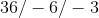Explanation:

We count the number of negative numbers. Any odd amount of negative numbers, our answer will be negative. Two is even so our answer is positive. Work from left to right.

Dividing the first two terms,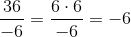now the expression becomes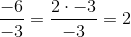.

Answer is.

### Example Question #62 : Negative Numbers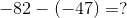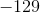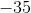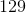Explanation:

When subtracting negative numbers, the two negative signs transform into an addition sign. Rewrite the question as follows to solve: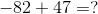To make the next step even easier, you may switch the position of each number in the sequence (only apply this to addition and subtraction problems):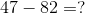As you can see, rewriting the question this way will alert you that the answer should be negative (since you are subtracting a bigger number from a smaller number). One last step to make solving this question even easier is to rewrite the question like this: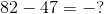### Example Question #63 : Negative Numbers

Solve: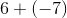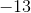Explanation:

When a plus and minus sign meet, we have a minus sign. Sinceis greater thanand is negative, our answer is negative. We treat as a normal subtraction. The answer is.

### Example Question #11 : Understand Distances Between Numbers On A Number Line: Ccss.Math.Content.7.Ns.A.1b

Solve: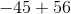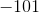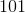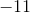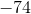Explanation:

Sinceis greater thanand is positive, our answer is positive. We treat as a normal subtraction. The answer is.

### Example Question #51 : The Number System

Solve: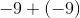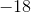Explanation:

When adding two negative numbers, we treat as an addition problem and add the minus sign in the end. The answer is.

### Example Question #51 : The Number System

Solve: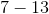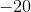Explanation:

Sinceis greater thanand is negative, our answer is negative. We treat as a normal subtraction. Answer is.

### Example Question #1 : Subtract Rational Numbers And Understand The Absolute Value Of Their Difference: Ccss.Math.Content.7.Ns.A.1c

Solve: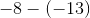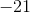Explanation:

Two minus signs becomes a plus sign. Sinceis greater thanand is positive, our answer is positive. We treat as a normal subtraction. Answer is.

### Example Question #71 : The Number System

Solve: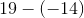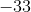Explanation:

Two minus signs become a plus sign. This is just simple addition. Answer is.

### Example Question #1 : Interpret Products Of Rational Numbers And Understand Properites Of Operations: Ccss.Math.Content.7.Ns.A.2a

Solve: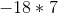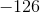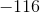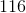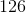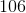Explanation:

When multiplying a negative number and a positive number, our answer is negative. Multiply normally. Answer is.

### Example Question #281 : Operations And Properties

Solve: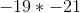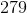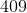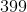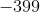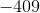When multiplying two negative numbers, the answer is positive. Then multiply normally. Answer is.